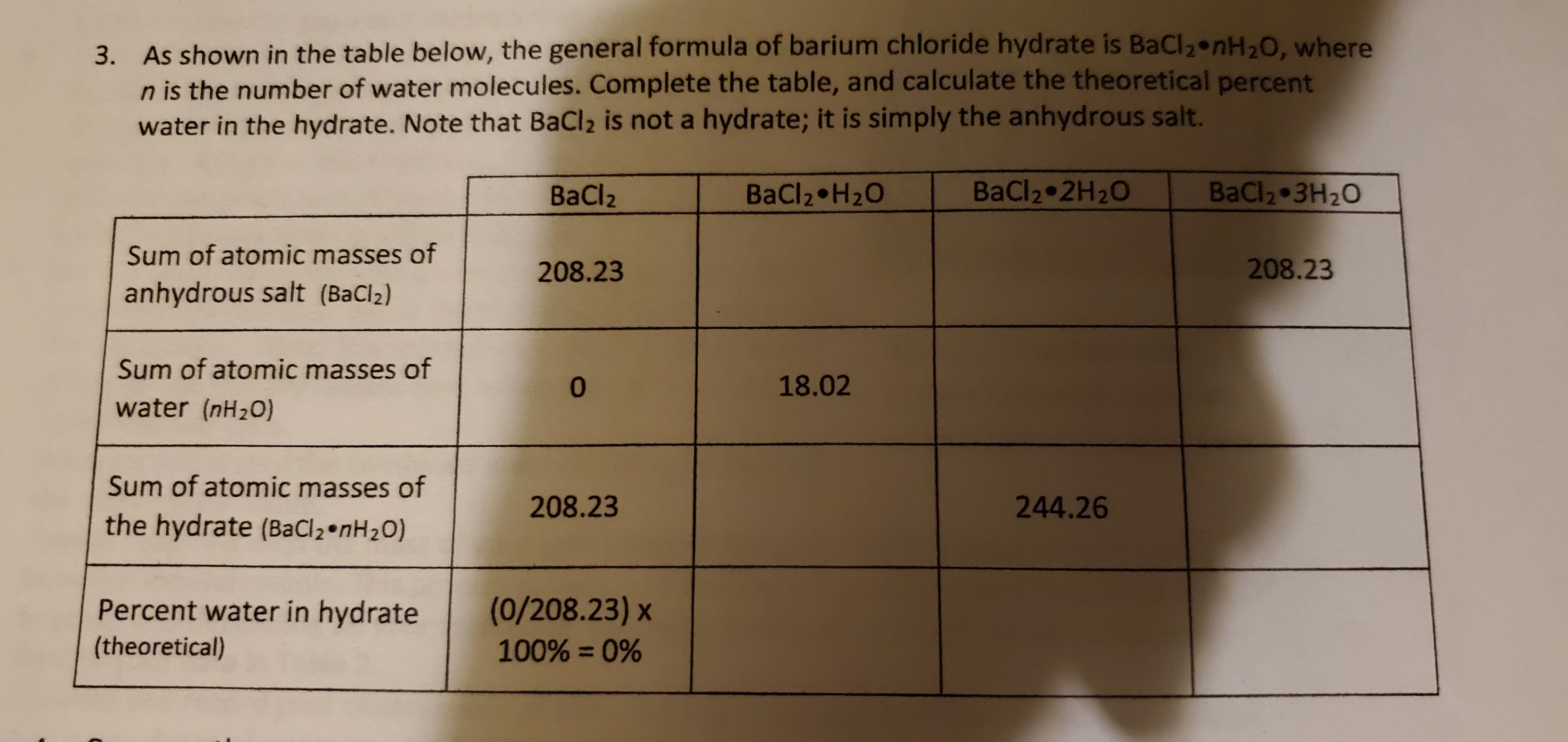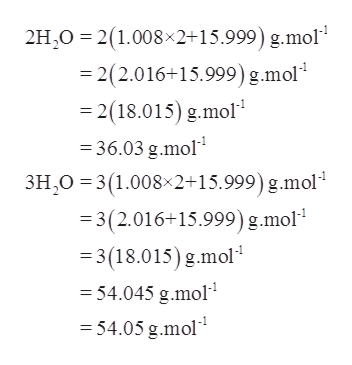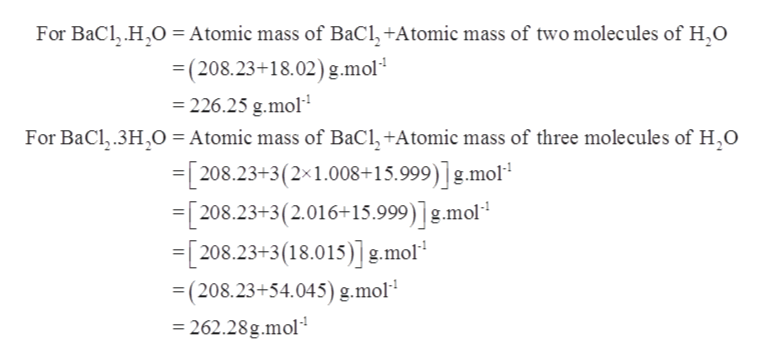# 3. As shown in the table below, the general formula of barium chloride hydrate is BaCl2 nH20, wheren is the number of water molecules. Complete the table, and calculate the theoretical percentwater in the hydrate. Note that BaCl2 is not a hydrate; it is simply the anhydrous salt.BaCl2BaCl2 H20BaCl2 2H20BaCl2 3H20Sum of atomic masses of208.23208.23anhydrous salt (BaCl2)Sum of atomic masses of018.02water (nH20)Sum of atomic masses of208.23244.26the hydrate (Ba Cl 2 *n H2 O)(0/208.23) x100%= 0%Percent water in hydrate(theoretical)

Question
348 views

Don't get how to fill in the table attached below.help_outlineImage Transcriptionclose3. As shown in the table below, the general formula of barium chloride hydrate is BaCl2 nH20, where n is the number of water molecules. Complete the table, and calculate the theoretical percent water in the hydrate. Note that BaCl2 is not a hydrate; it is simply the anhydrous salt. BaCl2 BaCl2 H20 BaCl2 2H20 BaCl2 3H20 Sum of atomic masses of 208.23 208.23 anhydrous salt (BaCl2) Sum of atomic masses of 0 18.02 water (nH20) Sum of atomic masses of 208.23 244.26 the hydrate (Ba Cl 2 *n H2 O) (0/208.23) x 100%= 0% Percent water in hydrate (theoretical) fullscreen
check_circle

Step 1

The number of water molecules present inside a crystal is known as water of crystallization.

The salts in which the compound is attached to some water of crystallization are known as hydrated salts. For example, copper sulphate is a hydrated salt having 5 molecules of water of crystallization which is written as CuSO4.5H2O.

On the other hand, salts which loses their water of crystallization on heating are known as anhydrous salts. For example, Barium chloride

Step 2

Atomic mass of anhydrous barium chloride = 208.23 g/mol.

Atomic mass of hydrogen = 1.008 u

Atomic mass of oxygen = 15.999 u

Therefore, sum of atomic masses of water that is 2H2O and 2H2O respectively will be calculated as follows:help_outlineImage Transcriptionclose2H,O 2(1.008x2+15.999) g.mol - 2(2.0 16+15.999) g.mol - 2(18.015) g.mol 36.03 g.mol 3H O 3(1.008 2+15.999) g.mol =3(2.016+15.999) g.mol 3(18.015) g.mol 54.045 g.mol 54.05 g.mol fullscreen
Step 3

The sum of atomic masses of hydrated that BaCl2.nH2O will b...help_outlineImage TranscriptioncloseFor BaCl, HO Atomic mass of BaCl,+Atomic mass of two molecules of H,O (208.23+18.02) g.mol = 226.25 g.mol For BaCl.3HO= Atomic mass of BaCl,+Atomic mass of three molecules of H,O =[208.23+3(2x1.008+15.999)] g.mol =[208.23+3(2.016+15.999)] g.mol =[208.23+3 (18.015)] g.mol (208.23+54.045) g.mol =262.28g.mol fullscreen

### Want to see the full answer?

See Solution

#### Want to see this answer and more?

Solutions are written by subject experts who are available 24/7. Questions are typically answered within 1 hour.*

See Solution
*Response times may vary by subject and question.
Tagged in

### Chemistry